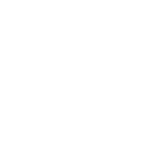Gardenvisit.com The Garden Guide

# The scales of Fahrenheit and Reaumur thermometers

1444. The scales of different thermometers are as follow:-In Fahrenheit's the freezing point is 32 degrees, and the boiling point 212 degrees. In Reaumur's, the freezing point is 0, and the boiling point 80 degrees. In the centigrade thermometer, which is generally used in France, and is the same as that of Celsius, which is the thermometer of Sweden, the freezing point is 0, and the boiling point 100 degrees. As a rule for comparing or reducing these scales, it may be stated that 1 degree of Reaumur's scale contains 2.5 degrees of Fahrenheit; and to convert the degrees of the one to the other, the rule is to multiply by 9, divide by 84, and add 32. One degree of the centigrade scale is equal to one degree and eight tenths of Fahrenheit; and the rule here is, to multiply by 9, divide by 5, and add 32. Any of these thermometers may be proved by immersing it in pounded ice for the freezing point, and in boiling water for the boiling point; and if the space, between these points is equally divided, the thermometer is correct.